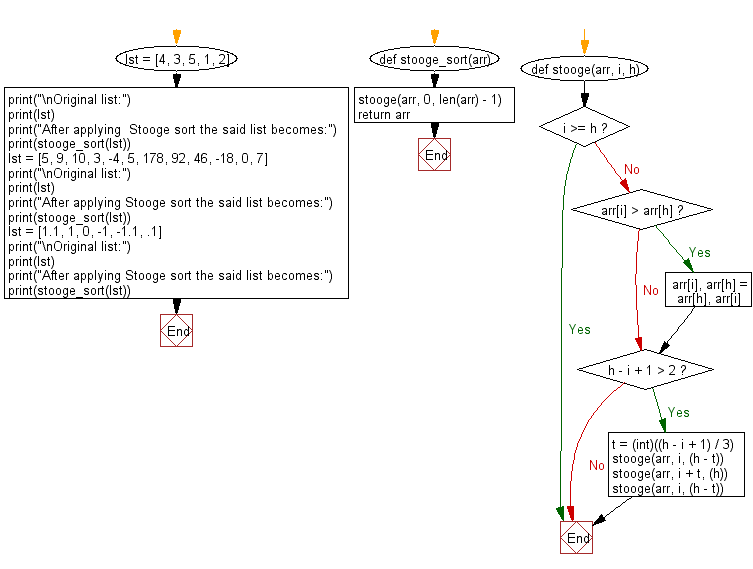﻿ Python: Sort unsorted numbers using Stooge sort - w3resource# Python: Sort unsorted numbers using Stooge sort

## Python Search and Sorting : Exercise-27 with Solution

Write a Python program to sort unsorted numbers using Stooge sort.

Stooge sort is a recursive sorting algorithm. It is notable for its exceptionally bad time complexity of O(nlog 3 / log 1.5 ) = O(n2.7095...). The running time of the algorithm is thus slower compared to reasonable sorting algorithms, and is slower than Bubble sort, a canonical example of a fairly inefficient sort. It is however more efficient than Slowsort. The name comes from The Three Stooges.
The algorithm is defined as follows:
• If the value at the start is larger than the value at the end, swap them.
• If there are 3 or more elements in the list, then:
Stooge sort the initial 2/3 of the list
Stooge sort the final 2/3 of the list

Sample Solution:

Python Code:

``````#Ref.https://bit.ly/3pk7iPH
def stooge_sort(arr):
stooge(arr, 0, len(arr) - 1)
return arr
def stooge(arr, i, h):
if i >= h:
return
# If first element is smaller than the last then swap them
if arr[i] > arr[h]:
arr[i], arr[h] = arr[h], arr[i]
# If there are more than 2 elements in the array
if h - i + 1 > 2:
t = (int)((h - i + 1) / 3)
# Recursively sort first 2/3 elements
stooge(arr, i, (h - t))
# Recursively sort last 2/3 elements
stooge(arr, i + t, (h))
# Recursively sort first 2/3 elements
stooge(arr, i, (h - t))
lst = [4, 3, 5, 1, 2]
print("\nOriginal list:")
print(lst)
print("After applying  Stooge sort the said list becomes:")
print(stooge_sort(lst))
lst = [5, 9, 10, 3, -4, 5, 178, 92, 46, -18, 0, 7]
print("\nOriginal list:")
print(lst)
print("After applying Stooge sort the said list becomes:")
print(stooge_sort(lst))
lst = [1.1, 1, 0, -1, -1.1, .1]
print("\nOriginal list:")
print(lst)
print("After applying Stooge sort the said list becomes:")
print(stooge_sort(lst))
``````

Sample Output:

```Original list:
[4, 3, 5, 1, 2]
After applying  Stooge sort the said list becomes:
[1, 2, 3, 4, 5]

Original list:
[5, 9, 10, 3, -4, 5, 178, 92, 46, -18, 0, 7]
After applying Stooge sort the said list becomes:
[-18, -4, 0, 3, 5, 5, 7, 9, 10, 46, 92, 178]

Original list:
[1.1, 1, 0, -1, -1.1, 0.1]
After applying Stooge sort the said list becomes:
[-1.1, -1, 0, 0.1, 1, 1.1]
```

Flowchart:Python Code Editor:

What is the difficulty level of this exercise?

Test your Programming skills with w3resource's quiz.

﻿

## Python: Tips of the Day

Use Reversed() In for Loops:

```>>> tasks = ['laundry', 'picking up kids', 'gardening', 'cooking']